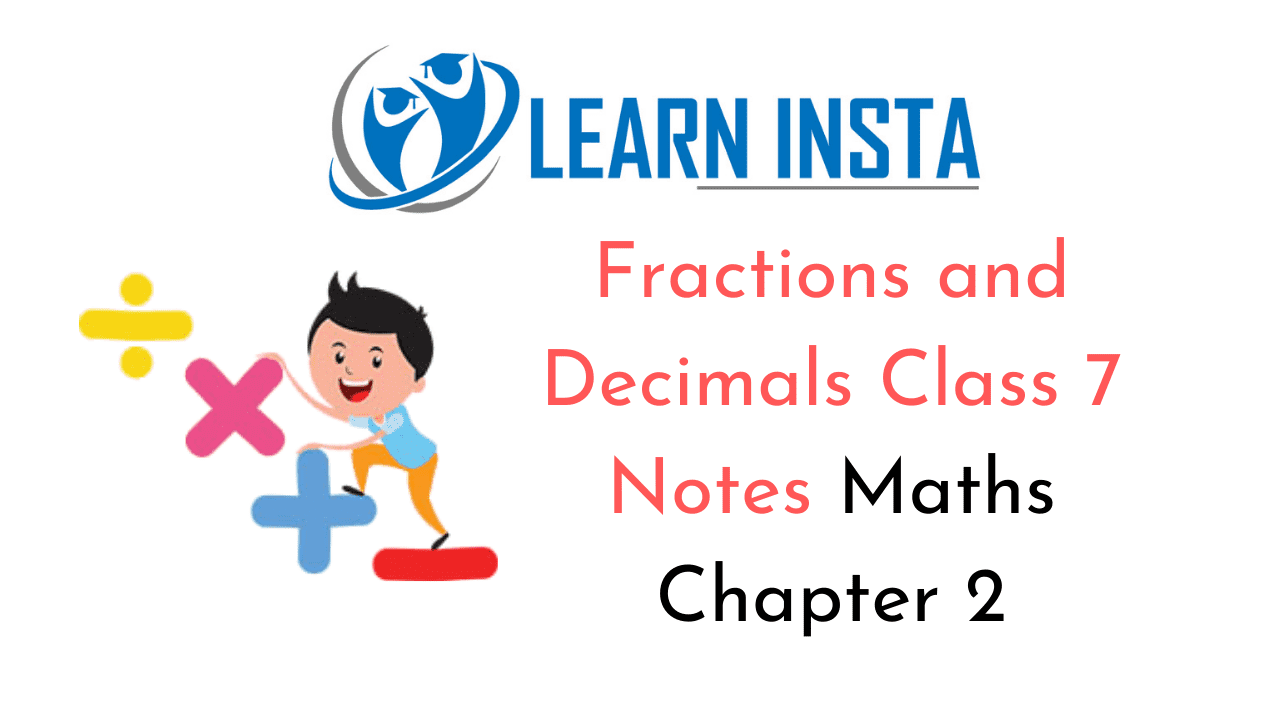On this page, you will find Fractions and Decimals Class 7 Notes Maths Chapter 2 Pdf free download. CBSE NCERT Class 7 Maths Notes Chapter 2 Integers will seemingly help them to revise the important concepts in less time.

## CBSE Class 7 Maths Chapter 2 Notes Fractions and Decimals

### Fractions and Decimals Class 7 Notes Conceptual Facts

Fraction $$=\frac{\text { Numerator }}{\text { Denominator }}$$

1. Types of fraction:

• Proper fractions = Representing a part of a whole, e.g$$\frac{1}{2}, \frac{3}{4} \text { and } \frac{1}{4}$$
• Improper fraction: Numerator is always greater than the denominator, $$\text { e.g. } \frac{6}{5}, \frac{7}{4} \text { and } \frac{3}{2}$$
• Mixed fraction: Combination of a whole number and a proper fraction.$$\text { -e.g. } 1 \frac{2}{3}, 2 \frac{5}{6} \text { and } 3 \frac{1}{2}$$

2. Multiplication of two fractions: $$\frac{\text { Product of numerators }}{\text { Product of denominators }} \text { e.g. } \frac{2}{5} \times \frac{3}{4}=\frac{2 \times 3}{5 \times 4}=\frac{6}{20}$$

3. Reciprocal of a non-zero fraction is obtained by interchanging its numerator and the denominator e.g. reciprocal of  $$\frac{3}{4} \text { is } \frac{4}{3}$$

4. While dividing a whole number by a fraction, we multiply the whole number with the reciprocal of that
$$\text { e.g. } 5 \div \frac{3}{2}=5 \times \frac{2}{3}=\frac{10}{3}$$

5. While dividing a fraction by a whole number, we multiply the fractions by the reciprocal of the whole
$$\text { e.g. } \frac{1}{4} \div 2=\frac{1}{4} \times \frac{1}{2}=\frac{1}{8}$$

6. Division of a fraction by another fractions.

7. Multiplication of two decimal numbers: 2.25 x 1.2 = 2.700

8. Number obtained by dividing a non-zero integer by 0, is not defined, e.g. $$\text { e.g. } \frac{2}{0}, \frac{\frac{3}{2}}{0} \text { and } \frac{-5}{0}$$